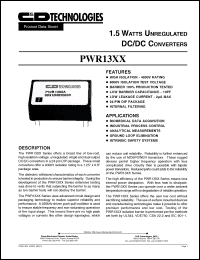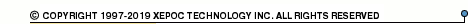More than478 245 registered clientsPWR1300 series datasheets. Manufacturer: CANDD.

 PWR1300 1.5 watt unregulated DC/DC converter. Nom.input voltage 5VDC, rated output voltage 5VDC, rated output current 300mA. in 24-pin DIP package. Operational temperature range from -40°C to 85°C. Datasheet*) PWR1301 1.5 watt unregulated DC/DC converter. Nom.input voltage 5VDC, rated output voltage 12VDC, rated output current 125mA. in 24-pin DIP package. Operational temperature range from -40°C to 85°C. Datasheet*) PWR1302 1.5 watt unregulated DC/DC converter. Nom.input voltage 5VDC, rated output voltage 15VDC, rated output current 100mA. in 24-pin DIP package. Operational temperature range from -40°C to 85°C. Datasheet*) PWR1303 1.5 watt unregulated DC/DC converter. Nom.input voltage 5VDC, rated output voltage +-5VDC, rated output current +-150mA. in 24-pin DIP package. Operational temperature range from -40°C to 85°C. Datasheet*) PWR1304 1.5 watt unregulated DC/DC converter. Nom.input voltage 5VDC, rated output voltage +-12VDC, rated output current +-63mA. in 24-pin DIP package. Operational temperature range from -40°C to 85°C. Datasheet*) PWR1305 1.5 watt unregulated DC/DC converter. Nom.input voltage 5VDC, rated output voltage +-15VDC, rated output current +-50mA. in 24-pin DIP package. Operational temperature range from -40°C to 85°C. Datasheet*) PWR1306 1.5 watt unregulated DC/DC converter. Nom.input voltage 12VDC, rated output voltage 5VDC, rated output current 300mA. in 24-pin DIP package. Operational temperature range from -40°C to 85°C. Datasheet*) PWR1307 1.5 watt unregulated DC/DC converter. Nom.input voltage 12VDC, rated output voltage 12VDC, rated output current 125mA. in 24-pin DIP package. Operational temperature range from -40°C to 85°C. Datasheet*) PWR1308 1.5 watt unregulated DC/DC converter. Nom.input voltage 12VDC, rated output voltage 15VDC, rated output current 100mA. in 24-pin DIP package. Operational temperature range from -40°C to 85°C. Datasheet*) PWR1309 1.5 watt unregulated DC/DC converter. Nom.input voltage 12VDC, rated output voltage +-5VDC, rated output current +-150mA. in 24-pin DIP package. Operational temperature range from -40°C to 85°C. Datasheet*) PWR1310 1.5 watt unregulated DC/DC converter. Nom.input voltage 12VDC, rated output voltage +-12VDC, rated output current +-63mA. in 24-pin DIP package. Operational temperature range from -40°C to 85°C. Datasheet*) PWR1311 1.5 watt unregulated DC/DC converter. Nom.input voltage 12VDC, rated output voltage +-15VDC, rated output current +-50mA. in 24-pin DIP package. Operational temperature range from -40°C to 85°C. Datasheet*) PWR1312 1.5 watt unregulated DC/DC converter. Nom.input voltage 15VDC, rated output voltage 5VDC, rated output current 300mA. in 24-pin DIP package. Operational temperature range from -40°C to 85°C. Datasheet*) PWR1313 1.5 watt unregulated DC/DC converter. Nom.input voltage 15VDC, rated output voltage 12VDC, rated output current 125mA. in 24-pin DIP package. Operational temperature range from -40°C to 85°C. Datasheet*) PWR1314 1.5 watt unregulated DC/DC converter. Nom.input voltage 15VDC, rated output voltage 15VDC, rated output current 100mA. in 24-pin DIP package. Operational temperature range from -40°C to 85°C. Datasheet*) PWR1315 1.5 watt unregulated DC/DC converter. Nom.input voltage 15VDC, rated output voltage +-5VDC, rated output current +-150mA. in 24-pin DIP package. Operational temperature range from -40°C to 85°C. Datasheet*) PWR1316 1.5 watt unregulated DC/DC converter. Nom.input voltage 15VDC, rated output voltage +-12VDC, rated output current +-63mA. in 24-pin DIP package. Operational temperature range from -40°C to 85°C. Datasheet*) PWR1317 1.5 watt unregulated DC/DC converter. Nom.input voltage 15VDC, rated output voltage +-15VDC, rated output current +-50mA. in 24-pin DIP package. Operational temperature range from -40°C to 85°C. Datasheet*)